# Overview:

 Three interrelated topics at the heart of logic and computer science.
 The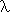-Calculus A pure calculus of functional abstraction and function application, with applications throughout logic and computer science. Types The-calculus is good tool for exploring type systems, invaluable both in the foundations of mathematics and for practical programming languages. Pure Type Systems A further generalisation and systematic presentation of the class of type systems found in the-cube.
 Combinators Combinatory logic shows that bound variables can be eliminated without loss of expressiveness. It has applications both in the foundations of mathematics and in the implementation of functional programming languages. Programming Languages The connections between the lambda-calculus and programming languages are diverse and pervasive. Type systems are an important aspect of programming language design.
 The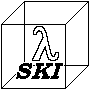-cube A graphical presentation of the relationship between combinatory logic, lambda calculi and related logical systems. The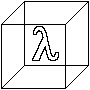-cube A graphical presentation of the relationship between various typed-calculi, illuminating the structure of Coquand's Calculus of Constructions.

# The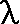-calculus

 A pure calculus of functional abstraction and function application, with applications throughout logic and computer science.

# Combinators

 Combinatory logic shows that bound variables can be eliminated without loss of expressiveness. It has applications both in the foundations of mathematics and in the implementation of functional programming languages.

Combinatory logic was invented by Moses Shönfinkel in 1920. The work was published in 1924 in a paper entitled On the building blocks of mathematical logic.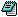At this time reducing logic to the simplest possible primitive basis was still thought to be worthwhile (it is not so greatly valued today). Schönfinkel showed how the use of bound variables in logic could be dispensed with. The use of "curried" higher order functions makes possible the reduction of logic to a language consisting of one constructor (the application of a function to an argument) and three primitive constants U, C (now usually called K) and S.

Schönfinkel's paper remains an accessible introduction to combinatory logic which makes clear the original motivation for this innovation. This original motivation was vigorously pursued later by H.B.Curry and his collaborators. The results of Curry's programme were published in Combinatory Logic, Vols 1 & 2.

While Schöfinkel was successful in showing that combinators could provide plausible notation, the semantic and proof theoretic aspects were more difficult to put in place. The difficulty is more pronounced if it hoped to use combinatory logic as a foundation for mathematics, than it is for the use of combinators in less demanding roles (e.g. for programming languages).

The Pure combinatory logic may be thought of as Schönfinkels system with the logical operator "U" omitted (though the presentation may vary). Illative combinatory logics are formed by adding additional logical primitives to the pure combinators. There are many illative combinatory logics, which vary among themselves in substance as well as in presentation.

Pure combinatory logic is so closely related to Church's lambda-calculus that it is best studied alongside the lambda-calculus, for which the most comprehensive modern text is probably The Lambda Calculus. A very popular and entertaining introduction to the pure combinators may be found in To Mock a Mockingbird.

Research on illative combinatory logics has been continued by Curry's students, Roger Hindley and Jonathan Seldin, and by Martin Bunder. Hindley, Lercher and Seldin published An Introduction to Combinatory Logic, an excellent and readable short introduction to the subject, now superseded by the more comprehensive An Introduction to Combinators and the Lambda-Calculus

Recently Randall Holmes has developed logical systems based on combinatory logic and the lambda calculus using methods derived from Quine's formulations of set theory.

The attempt to make a foundation for mathematics using the illative combinatory logic were blighted by Curry's paradox and semantic opacity. While systems avoiding this paradox have been devised, they are typically weak, and lack the kind of convincing semantic intuition found in first order set theory.

# Types

 The-calculus is good tool for exploring type systems, invaluable both in the foundations of mathematics and for practical programming languages.

# The-SKI Cube

 The-SKI cube is a way of presenting a number of related calculi and logics which are obtainable from pure combinatory logic by one or more of three kinds of extension. The origin of the cube is pure Combinatory Logic (c). The three kinds of extension considered are called the axes of the cube. The cube presents eight systems or kinds of system which are arranged at the vertices of the cube. Those systems sharing a particular value on one of the dimensions of the cube are arranged around one of the faces of the cube.

# The-cube

 Thecube is a way of presenting the fine structure of Coquand's Calculus of Constructions relating that system to a variety of other typed lambda calculi. This idea is due to Henk Barendregt, and is presented in his Introduction to Generalised Type Systems which is my principle source in preparing this material. The eight systems at the vertices of the cube are obtained by extending the simply typed lambda calculus (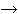) at the origin of the cube along one or more of the axes of the cube. The cube has six faces (like they do).

#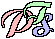Pure Type Systems - distilling an essence of type assignment for the lambda calculus.
Pure type systems are a family of typed lambda calculi each member of which is determined by a triple (S,A,R) where:
S is a subset of the constants of the system known as the sorts is a set of axioms of the form: c:s, where c is a constant and s is a sort. is a set of triples of sorts which determines what function spaces can be constructed in the system and what sort each function space inhabits
Each PTS is a formal system in which judgements of the form:

ContextTypeAssignment

may be derived. These judgements assign a type to some term of the typed lambda calculus in the given context.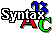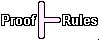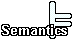# Programming Languages

 The connections between the lambda-calculus and programming languages are diverse and pervasive. Type systems are an important aspect of programming language design.
 LISP The list processing language LISP devised by John McCarthy in the late 1950's. LISP was the first of many functional programming languages which are closely related to the lambda calculus. It was and remains the dominant language for programming in the field of Artificial Intelligence. Computability The lambda calculus was first devised by Alonzo Church, first to provide a foundation for mathematics and then to show the existence of unsolvable problems. This lead to the development of a major branch of mathematical logic, the theory of computation, which inter alia, gives us via Church's Thesis (essentially that anything which can be computed can be computed using the lambda calculus) a grip on the limits in principle of what we can program computers to do.
 ML The language ML (for MetaLanguage) was developed at Edinburgh by Robin Milner et.al. for use in the development of proof tools. It incorporated a polymorphic strict type system which owes its origin to work on the lambda calculus. Combinator Graphs The connection between the lambda calculus and pure combinatory logic was exploited to yield efficient techniques for the evaluation of functional programs by the reduction of graphs of combinators. These techniques were popular in the 1980's, used notably by David Turner in implementations of his pure functional language Miranda. Formal Semantics Work on the semantics of programming languages, notably that on denotational semantics, has made use of the lambda calculus, which has also provided a testbed for some of this work.
 Programs from Proofs The constructive type theories devised by the philosopher per Martin Löf influenced work on the design of programming languages and on the verification of programs. By opening out the expressiveness of type systems by the introduction of "dependent types" they made it possible for types to be used as specifications for programs. Through the Propositions as Types metaphor it became possible to extract correct functional programs from proofs (of the propositions corresponding to their specifications). Type Systems It has now almost taken for granted that a programming language (whether functional or imperative) should have a type system, and devising the type system is an important early part of the design of the language. There is a rich literature on type systems which can be drawn on by language designers.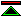©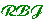created 1998/7/28 modified 1999/9/30